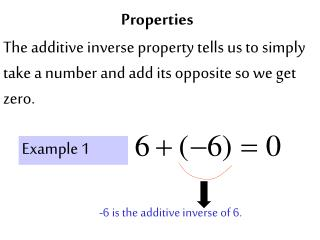# Properties - PowerPoint PPT PresentationDownload PresentationProperties

PropertiesDownload Presentation## Properties

- - - - - - - - - - - - - - - - - - - - - - - - - - - E N D - - - - - - - - - - - - - - - - - - - - - - - - - - -
##### Presentation Transcript

1. Properties The additive inverse property tells us to simply take a number and add its opposite so we get zero. Example 1 -6 is the additive inverse of 6.

2. Properties Example 2 The multiplicative inverse is when you take a number and multiply it by its reciprocal so you get one. So, is the multiplicative inverse of -5.

3. Properties Example 3 Identity Properties Very simple, the identity of addition is zero, and the identity of multiplication is one. Notice the identities give you back the number you started with.

4. Properties Identify and label the following properties. A. B. C.

5. Properties Properties Identify and label the following properties. Identify and label the following properties. A. B. C.

6. Properties Identify the property given. a. b. c.

7. Distributive property.

8. THE END